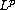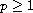Electron. J. Diff. Equ., Vol. 2015 (2015), No. 162, pp. 1-14.

### Estimates for damped fractional wave equations and applications Jiecheng Chen, Dashan Fan, Chunjie Zhang

Abstract:
In our previous article  we estimated the-norm () of the solution to damped fractional wave equation. In this article, we prove otherestimates, with some emphasis on requiring less regularity of the initial data. We also study the Strichartz type estimate of this equation. Finally we present some application of these estimates, for proving existence of global solutions to semilinear damped fractional wave equations.

Submitted November 30, 2014. Published June 16, 2015.
Math Subject Classifications: 35L05, 46E35, 42B37.
Key Words: Damped fractional wave equation;-estimate; Strichartz estimate.

Show me the PDF file (263 KB), TEX file, and other files for this article.Jiecheng Chen Department of Mathematics Zhejiang Normal University Jinhua 321004, China email: jcchen@zjnu.edu.cn Dashan Fan Department of Mathematics University of Wisconsin Milwaukee, WI 53201, USA email: fan@uwm.edu Chunjie Zhang Department of Mathematics Hangzhou Dianzi University Hangzhou 310018, China email: purezhang@hdu.edu.cn